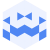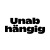Am 11. Apr. 2023 | 19:45 Uhr

# BNSU | Tresor Linz | April 11 2023 I canceled but not canceled ICreated at 26. Apr. 2023

86 Ansichten
##### by Tresor Linz

Tresor Linz - recorded on April 11 2023

<<<<<<<<<<<<<<<<<<<<<<<<<<<<<<<<<<<<<<<<<<<<<<<<<<<<<<<<<

BNSU
<<<<<<<<<<<<<<<<<<<<<<<<<<<<<<<<<<<<<<<<<<<<<<<<<<<<<<<<<

BNSU focuses on high impact, physical and extreme sounds; a mashup of all the -core genres turned up to eleven, a fluid, genre-hopping amalgamation of various styles of instigating a mosh pit.

<<<<<<<<<<<<<<<<<<<<<<<<<<<<<<<<<<<<<<<<<<<<<<<<<<<<<<<<<
Tresor Linz
<<<<<<<<<<<<<<<<<<<<<<<<<<<<<<<<<<<<<<<<<<<<<<<<<<<<<<<<<

Raum für Klangkunst und künstlerische Experimente.

Space for sound art and artistic experiments.

##### Share & Embed
Embed this Video